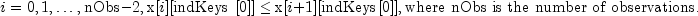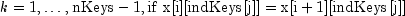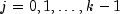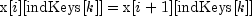JMSLTM Numerical Library 7.2.0
com.imsl.stat

## Class Sort

• ```public class Sort
extends Object```
A collection of sorting functions.

Class `Sort` contains ascending and descending methods for sorting elements of an array or a matrix.

The QuickSort algorithm is used, except for short sequences that are handled using an insertion sort.

The QuickSort algorithm is a randomized QuickSort with 3-way partitioning. Basic QuickSort is slow if the sequence to be sorted contains many duplicate keys. The 3-way partitioning algorithm eliminates this problem. The pivot is chosen as the middle element of three potential pivots chosen at random.

The matrix ascending method sorts the rows of real matrix `x` using a particular column or columns in `x` as the keys. The sort is algebraic with the first key as the most significant, the second key as the next most significant, etc. When x is sorted in ascending order, the resulting sorted array is such that the following is true:

• For• Forfor, thenThe observations also can be sorted in descending order.

The rows of `x` containing the missing value code NaN in at least one of the specified columns are considered as an additional group. These rows are moved to the end of the sorted `x`.

If all of the sort keys in a pair of rows are equal then the rows keep their original relative order.

The sorting algorithm is based on a quicksort method given by Singleton (1969) with modifications, see Bentley and Sedgewick (1997).

Example 1: Array sort with permutation vector, Example 2: Matrix sort containing NaN's
• ### Method Summary

Methods
Modifier and Type Method and Description
`static void` `ascending(double[] ra)`
Sorts an array into ascending order.
`static void` ```ascending(double[][] ra, int nKeys)```
Sorts a matrix into ascending order by the first `nKeys`.
`static void` ```ascending(double[][] ra, int[] indKeys)```
Sorts a matrix into ascending order by specified keys.
`static void` ```ascending(double[][] ra, int[] indKeys, int[] iPerm)```
Sorts a matrix into ascending order by specified keys and returns the permutation vector.
`static void` ```ascending(double[][] ra, int nKeys, int[] iPerm)```
Sorts a matrix into ascending order according to the first `nKeys` keys and returns the permutation vector.
`static void` ```ascending(double[] ra, int[] iPerm)```
Sorts an array into ascending order and returns the permutation vector.
`static void` `ascending(int[] ia)`
Sorts an integer array into ascending order.
`static void` ```ascending(int[][] ia, int nKeys)```
Sorts a matrix into ascending order by the first `nKeys`.
`static void` ```ascending(int[][] ia, int[] indKeys, int[] iPerm)```
Sorts a matrix into ascending order by specified keys and returns the permutation vector.
`static void` ```ascending(int[][] ia, int nKeys, int[] iPerm)```
Sorts a matrix into ascending order according to the first `nKeys` keys and returns the permutation vector.
`static void` ```ascending(int[] ia, int[] iPerm)```
Sorts an integer array into ascending order and returns the permutation vector.
`static void` `descending(double[] ra)`
Sorts an array into descending order.
`static void` ```descending(double[][] ra, int nKeys)```
Sorts a matrix into descending order by the first `nkeys`.
`static void` ```descending(double[][] ra, int[] indKeys)```
Sorts a matrix into descending order by specified keys.
`static void` ```descending(double[][] ra, int[] indKeys, int[] iPerm)```
Sorts a matrix into descending order by specified keys and return the permutation vector.
`static void` ```descending(double[][] ra, int nKeys, int[] iPerm)```
Sorts a matrix into descending order by the first `nkeys` and returns the permutation vector.
`static void` ```descending(double[] ra, int[] iPerm)```
Sorts an array into descending order and returns the permutation vector.
`static void` `descending(int[] ra)`
Sorts an integer array into descending order.
`static void` ```descending(int[] ra, int[] iPerm)```
Sorts an integer array into descending order and returns the permutation vector.
• ### Methods inherited from class java.lang.Object

`clone, equals, finalize, getClass, hashCode, notify, notifyAll, toString, wait, wait, wait`
• ### Method Detail

• #### ascending

`public static void ascending(double[] ra)`
Sorts an array into ascending order.
Parameters:
`ra` - a `double` array to be sorted into ascending order
• #### ascending

```public static void ascending(double[][] ra,
int nKeys)```
Sorts a matrix into ascending order by the first `nKeys`.
Parameters:
`ra` - a `double` matrix to be sorted into ascending order
`nKeys` - an `int`, the number of keys. The first `nKeys` columns of `ra` are to be used as the sorting keys.
• #### ascending

```public static void ascending(double[][] ra,
int[] indKeys)```
Sorts a matrix into ascending order by specified keys.
Parameters:
`ra` - a `double` matrix to be sorted into ascending order
`indKeys` - an `int` array containing the order into which the columns of `ra` are to be sorted. These values must be between 0 and one less than the number of columns in `ra`.
• #### ascending

```public static void ascending(double[][] ra,
int[] indKeys,
int[] iPerm)```
Sorts a matrix into ascending order by specified keys and returns the permutation vector.
Parameters:
`ra` - a `double` matrix to be sorted into ascending order
`indKeys` - an `int` array containing the order into which the columns of `ra` are to be sorted. These values must be between 0 and one less than the number of columns in `ra`.
`iPerm` - an `int` array that on input is the same length as `ra` and contains the values 0, 1, .... On output, the values represent the permutation of the values in `ra`, that is, the re-ordering of those values in terms of their previous position.
• #### ascending

```public static void ascending(double[][] ra,
int nKeys,
int[] iPerm)```
Sorts a matrix into ascending order according to the first `nKeys` keys and returns the permutation vector.
Parameters:
`ra` - a `double` matrix to be sorted into ascending order
`nKeys` - an `int`, the number of keys. The first `nKeys` columns of `ra` are to be used as the sorting keys.
`iPerm` - an `int` array that on input is the same length as `ra` and contains the values 0, 1, .... On output, the values represent the permutation of the values in `ra`, that is, the re-ordering of those values in terms of their previous position.
• #### ascending

```public static void ascending(double[] ra,
int[] iPerm)```
Sorts an array into ascending order and returns the permutation vector.
Parameters:
`ra` - a `double` array to be sorted into ascending order
`iPerm` - an `int` array that on input is the same length as `ra` and contains the values 0, 1, .... On output, the values represent the permutation of the values in `ra`, that is, the re-ordering of those values in terms of their previous position.
• #### ascending

`public static void ascending(int[] ia)`
Sorts an integer array into ascending order.
Parameters:
`ia` - an `int` array to be sorted into ascending order
• #### ascending

```public static void ascending(int[][] ia,
int nKeys)```
Sorts a matrix into ascending order by the first `nKeys`.
Parameters:
`ia` - an `int` matrix to be sorted into ascending order
`nKeys` - an `int`, the number of keys. The first `nKeys` columns of `ia` are to be used as the sorting keys.
• #### ascending

```public static void ascending(int[][] ia,
int[] indKeys,
int[] iPerm)```
Sorts a matrix into ascending order by specified keys and returns the permutation vector.
Parameters:
`ia` - an `int` matrix to be sorted into ascending order
`indKeys` - an `int` array containing the order into which the columns of `ia` are to be sorted. These values must be between 0 and one less than the number of columns in `ia`.
`iPerm` - an `int` array that on input is the same length as `ia` and contains the values 0, 1, .... On output, the values represent the permutation of the values in `ia`, that is, the re-ordering of those values in terms of their previous position.
• #### ascending

```public static void ascending(int[][] ia,
int nKeys,
int[] iPerm)```
Sorts a matrix into ascending order according to the first `nKeys` keys and returns the permutation vector.
Parameters:
`ia` - an `int` matrix to be sorted into ascending order
`nKeys` - an `int`, the number of keys. The first `nKeys` columns of `ia` are to be used as the sorting keys.
`iPerm` - an `int` array that on input is the same length as `ia` and contains the values 0, 1, .... On output, the values represent the permutation of the values in `ia`, that is, the re-ordering of those values in terms of their previous position.
• #### ascending

```public static void ascending(int[] ia,
int[] iPerm)```
Sorts an integer array into ascending order and returns the permutation vector.
Parameters:
`ia` - an `int` array to be sorted into ascending order
`iPerm` - an `int` array that on input is the same length as `ia` and contains the values 0, 1, .... On output, the values represent the permutation of the values in `ia`, that is, the re-ordering of those values in terms of their previous position.
• #### descending

`public static void descending(double[] ra)`
Sorts an array into descending order.
Parameters:
`ra` - a `double` array to be sorted into descending order
• #### descending

```public static void descending(double[][] ra,
int nKeys)```
Sorts a matrix into descending order by the first `nkeys`.
Parameters:
`ra` - a `double` matrix to be sorted into descending order
`nKeys` - an `int`, the number of keys. The first `nKeys` columns of `ra` are to be used as the sorting keys.
• #### descending

```public static void descending(double[][] ra,
int[] indKeys)```
Sorts a matrix into descending order by specified keys.
Parameters:
`ra` - a `double` matrix to be sorted into descending order
`indKeys` - an `int` array containing the order into which the columns of `ra` are to be sorted. These values must be between 0 and one less than the number of columns in `ra`.
• #### descending

```public static void descending(double[][] ra,
int[] indKeys,
int[] iPerm)```
Sorts a matrix into descending order by specified keys and return the permutation vector.
Parameters:
`ra` - a `double` matrix to be sorted into descending order
`indKeys` - an `int` array containing the order into which the columns of `ra` are to be sorted. These values must be between 0 and one less than the number of columns in `ra`.
`iPerm` - an `int` array that on input is the same length as `ra` and contains the values 0, 1, .... On output, the values represent the permutation of the values in `ra`, that is, the re-ordering of those values in terms of their previous position.
• #### descending

```public static void descending(double[][] ra,
int nKeys,
int[] iPerm)```
Sorts a matrix into descending order by the first `nkeys` and returns the permutation vector.
Parameters:
`ra` - a `double` matrix to be sorted into descending order
`nKeys` - an `int`, the number of keys. The first `nKeys` columns of `ra` are to be used as the sorting keys.
`iPerm` - an `int` array that on input is the same length as `ra` and contains the values 0, 1, .... On output, the values represent the permutation of the values in `ra`, that is, the re-ordering of those values in terms of their previous position.
• #### descending

```public static void descending(double[] ra,
int[] iPerm)```
Sorts an array into descending order and returns the permutation vector.
Parameters:
`ra` - a `double` array to be sorted into descending order
`iPerm` - an `int` array that on input is the same length as `ra` and contains the values 0, 1, .... On output, the values represent the permutation of the values in `ra`, that is, the re-ordering of those values in terms of their previous position.
• #### descending

`public static void descending(int[] ra)`
Sorts an integer array into descending order.
Parameters:
`ra` - an `int` array to be sorted into descending order
• #### descending

```public static void descending(int[] ra,
int[] iPerm)```
Sorts an integer array into descending order and returns the permutation vector.
Parameters:
`ra` - an `int` array to be sorted into descending order
`iPerm` - an `int` array that on input is the same length as `ra` and contains the values 0, 1, .... On output, the values represent the permutation of the values in `ra`, that is, the re-ordering of those values in terms of their previous position.
JMSLTM Numerical Library 7.2.0